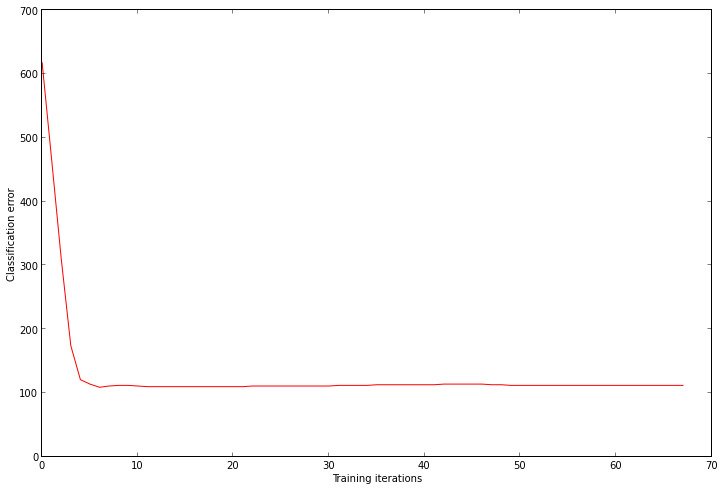# Autotelic Computing

## Monday, October 7, 2013

### Logistic Regression 101

As this seems to be becoming a series of related posts, see also:
1. Linear Regression 101
2. Logistic Regression 101
3. Softmax Regression 101
4. Neural Network 101

## A Cloud of Points¶

We will next tackle the problem of binary supervised classification, using the logistic regression method. The artificial dataset we will use this time is built by sampling random 2D points in the plane, and assigning them a class (0 or 1) with a probability that is proportional to their distance from a line with given slope and intercept parameters. In other words, if we consider a class 0 point on the left of the dividing line (and the same for class 1 on the right) as correctly classified, the points far from the line (on either side) are likely to be correctly classified, while those nearer are more likely to be misclassified. The goal will be to build a classifier able to distinguish the two classes for a given point, and use it to classify any new point which wasn't part of the training set.
In :
def dataset(n, slope, intercept):
xy = random.uniform(low=-2, high=2, size=(n, 2))
classes = []
for p in xy:
d = (p - slope * p - intercept) / sqrt(slope ** 2 + 1)
classes.append(0 if d < random.normal(scale=0.5) else 1)
return column_stack([xy, classes])

random.seed(0)
points = dataset(1000, 2.5, -1)
class0 = points[where(points[:,2] == 0)]
class1 = points[where(points[:,2] == 1)]
plot(class0[:,0], class0[:,1], 'bo')
plot(class1[:,0], class1[:,1], 'r^');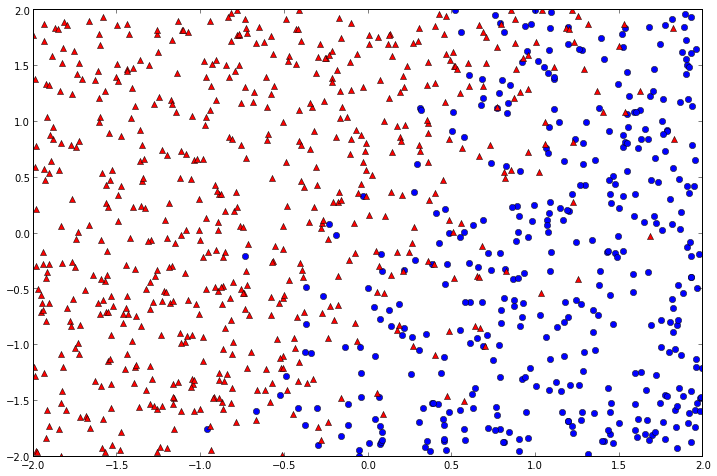## Guessing an Initial Model¶

As we did for linear regression, we first guess an initial set of $\theta$ parameters for our model. This time it has an extra component, since our inputs are characterized by two coordinates ($x$ and $y$), instead of one. It is still a linear model though, and $\theta$ can be interpreted as the parameters of an equation of the general form ($ax + by + c = 0$): $\theta_1 x + \theta_2 y + \theta_0 = 0$. We need a simple function to convert between the general and slope-intercept form, for drawing purposes, but of course they're equivalent.
In :
def general_to_slope_intercept(theta):
slope = -theta / theta
intercept = -theta / theta
return slope, intercept

initial_theta = random.sample(3)
slope, intercept = general_to_slope_intercept(initial_theta)
plot(class0[:,0], class0[:,1], 'bo')
plot(class1[:,0], class1[:,1], 'r^')
plot(linspace(-2, 2), slope * linspace(-2, 2) + intercept,
'r-', label='initial guess', linewidth=2)
axis((-2, 2, -2, 2))
legend();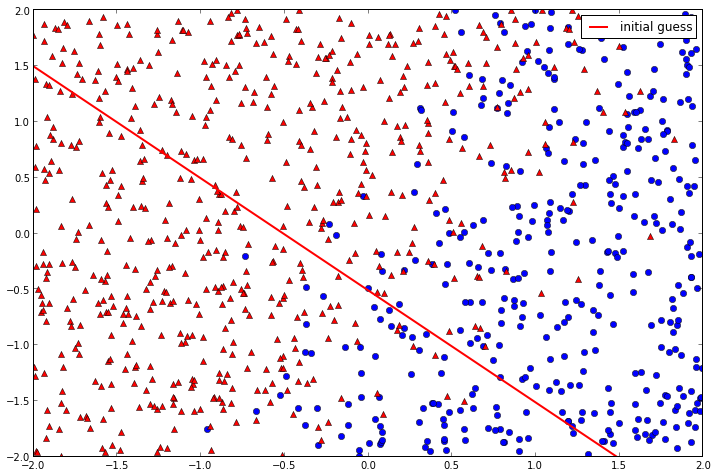## Introducing Probabilities¶

Even though the graphical representation we've been using may suggest otherwise, what we're really dealing with this time is class membership, and only indirectly planar position. And since class membership, our target variable, is binary, rather than scalar (as was the case with linear regression), one way to think about it is in terms of the probability, for a given point, to belong to a certain class
$P(C = k | \vec{x}; \theta)$
in other words, given its 2D coordinates (note the vector notation, to emphasize that $x$ here corresponds to 2D coordinates, not a scalar feature as with linear regression) and the current $\theta$ parameters, what's the probability for a certain point to be classified as $k$, where $k \in \{0,1\}$. This is what we'll try to model.
It turns out that there's a simple non-linear function that squashes its input into the range $[0, 1]$: the logistic function
$h(x) = \frac{1}{1 + e^{-x}}$
Although this function, in itself, has nothing to do with probabilities, there's nothing that prevent us from using it to endow our model with probabilistic semantics. In particular, we can turn the attributes of a point $\vec{x}$ into a probability estimate, just by passing its dot product with $\theta$ through the logistic function, which will squash it appropriately
$P(C = k | \vec{x}; \theta) = h(\theta^T \vec{x})$
In :
def logistic(x):
return 1 / (1 + exp(-x))

plot(linspace(-10, 10), logistic(linspace(-10, 10)), 'b-');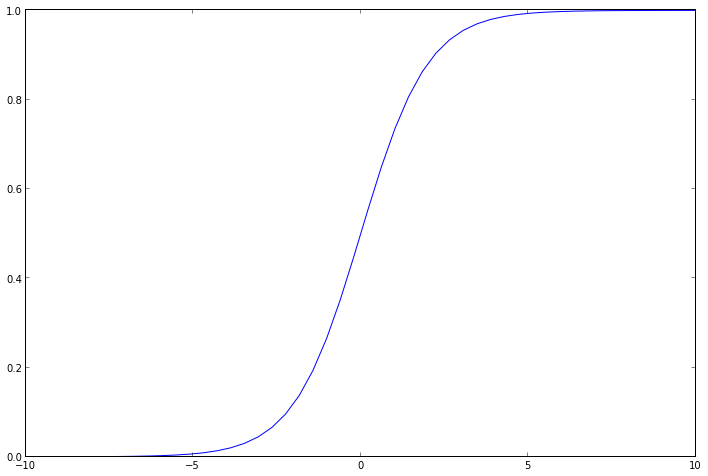## Training to Get Better¶

As with linear regression, we will find the best parameters for the model by minimizing an error function. This time however the function is probabilistic, and is called the negative log-likelihood
$NLL = - \sum_{i}^{n} c^{(i)} \log h_{\theta}(\vec{x}^{(i)}) + (1 - c^{(i)}) \log (1 - h_{\theta}(\vec{x}^{(i)})$
where $c^{(i)}$ is the class (0 or 1) of the $i$-th point, $\vec{x}^{(i)}$ its 2D coordinates, and $h_{\theta}$ denotes our model itself, consisting in the squashed dot product of the coordinates with its current state, $\theta$.
As with linear regression, we use the batch gradient descent method to minimize this function, by taking iterative steps in the direction of its derivative (gradient), which has a surprisingly familiar form
$\frac{\partial NLL}{\partial \theta_j} = (c - h_{\theta}(\vec{x})) \cdot \vec{x}_j$
Indeed the error function derivatives for both linear and logistic regressions are identical, and the reason for this is that they can be both thought as being specific instances of a broader class called generalized linear models. Following that, there's a way to reinterpret the MSE of the linear regression, also in probabilistic terms.
The training algorithm for logistic regression is thus very close to the one for linear regression, with the exception of the $h$ function, which is now logistic, and so introduces non-linearity in the model
$\theta_j := \theta_j - \alpha \sum_{i}^{n} (h_{\theta}(\vec{x}_j^{(i)}) - c^{(i)}) \cdot \vec{x}_j^{(i)}$
Despite this non-linearity, it is worth noting that the optimal decision boundary found by training is still a 2D line, as logistic regression is fundamentally a linear modeling method. In particular, when we apply the "general to slope-intercept" form transformation for the optimal $\theta$, we find approximate values of the original parameters that we used to generate the dataset.
In :
def train(points, initial_theta, alpha=0.001, stop=1e-4):
x, y, c = points[:,0], points[:,1], points[:,2]
n = len(points)
bxy = column_stack([ones(n), x, y])
thetas = [initial_theta]
nll_errors = []
classif_errors = []
while True:
theta = thetas[-1].copy()
h = logistic(dot(bxy, theta))
nll_errors.append(-sum(c * log(h) + (1 - c) * log(1 - h)) / n)
classif_errors.append(sum((np.round(h) != c).astype(int)))
if len(nll_errors) > 1 and nll_errors[-2] - nll_errors[-1] < stop: break
theta -= alpha * sum(h - c) # intercept component
theta -= alpha * sum((h - c) * x)
theta -= alpha * sum((h - c) * y)
thetas.append(theta)
return thetas, nll_errors, classif_errors

thetas, nll_errors, classif_errors = train(points, initial_theta)
best_theta = thetas[-1] # last one
plot(class0[:,0], class0[:,1], 'bo')
plot(class1[:,0], class1[:,1], 'r^')
slope, intercept = general_to_slope_intercept(best_theta)
print 'best_theta slope and intercept = %f, %f' % (slope, intercept)
plot(linspace(-2, 2), slope * linspace(-2, 2) + intercept,
'g-', label='trained model', linewidth=2)
axis((-2, 2, -2, 2))
legend();

best_theta slope and intercept = 2.546265, -1.042059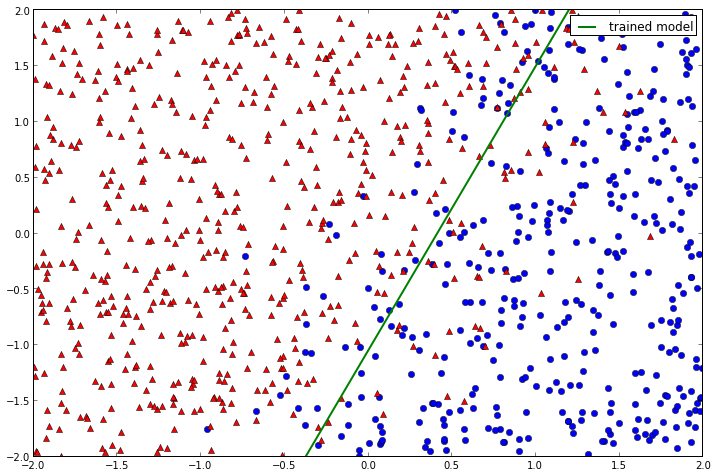We can plot the successive values of the NLL error to confirm that it was minimized.
In :
xlabel('Training iterations')
ylabel('NLL')
plot(range(len(nll_errors)), nll_errors, 'r-');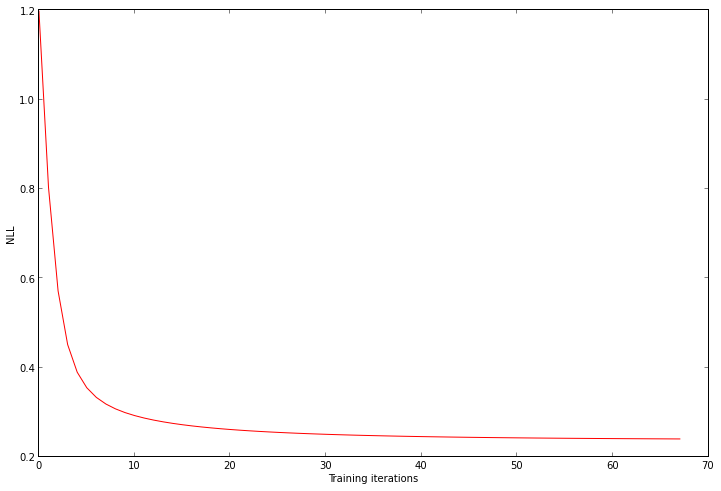But we can also look at the evolution of another error function, the classification error, which simply tracks, while training, the number of misclassified points. Although we're not directly minimizing this function, it can be thought as a proxy to the NLL, with which it is highly correlated. It is also more intuitive, and thus easier to interpret and make sense of. The interesting thing about this function in our case is that although it first steadily decreases, it rapidly stabilizes itself at around 100, meaning that no matter how hard we train, we always have many points that are not correctly classified. By looking at the dataset it is obvious that there is no line that could perfectly separate the two classes (we actually built the dataset that very way), and logistic regression simply does its best, finding the best compromise.
In :
xlabel('Training iterations')
ylabel('Classification error')
plot(range(len(classif_errors)), classif_errors, 'r-')
ylim(0);